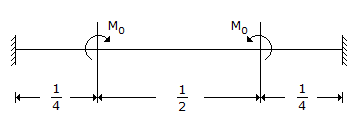# Civil Engineering - UPSC Civil Service Exam Questions

36.

A fixed beam is subjected to ment M0 as shown in the figure. The fixed end moments will be :A. zero B. M0 C. M0/2 D. 2M0

Explanation:

No answer description available for this question. Let us discuss.

37.

Consider the following steps :
1. Driving sheet piles surrounding a vibration-receiving structure.
2. Digging a trench around a source of vibration.
3. Placing rubber mountings between a machine causing vibration and its base. Active isolation of vibration can be achieved by

 A. 1 and 2 B. 1 and 3 C. 2 and 3 D. 3 alone

Explanation:

No answer description available for this question. Let us discuss.

38.

Two reservoirs at different surface elevations are connected by a set of two pipes A and B of same diameter and length in parallel. If the friction factor of A is 4 times that of the pipe B, what is the ratio of the discharge in A to that in B ?

 A. 0.25 B. 4 C. 2 D. 0.5

Explanation:

No answer description available for this question. Let us discuss.

39.

Which of the following should be employed to provide lateral support to the beams ?
1. Bracing of compression flanges.
2. Shear connectors.
3. Bracing of tension flanges
4. Embedding compression flanges into R.C.C. slab.
Select the correct answer using the codes given below :

 A. 1 only B. 1 and 4 C. 2 and 3 D. 1, 2 and 4